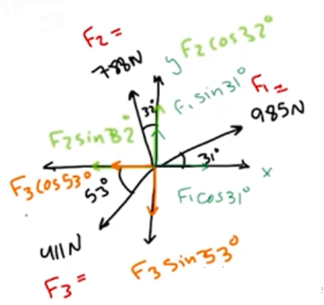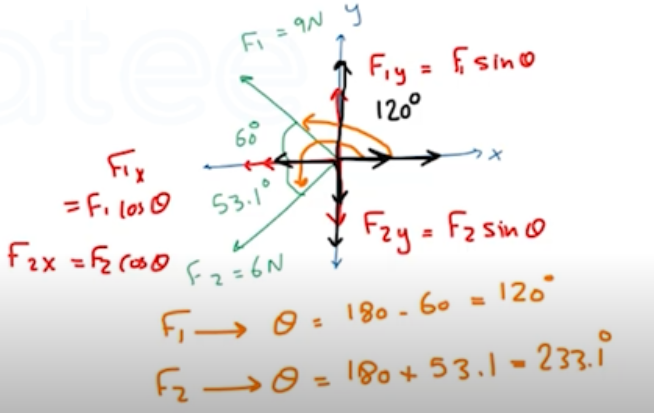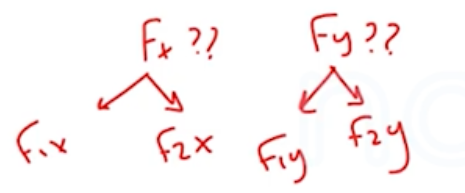Need Help?

Subscribe to Physics 1

###### \${selected_topic_name}
• Notes

$\begin{array}{l}{\text { Workmen are trying to free an SUV stuck in the mud. To extricate the vehicle, they use three horizontal }} \\ {\text { ropes, producing the force vectors. (a) Find the } x \text { -and } y \text { -components of each of the three pulls. (b) Use }} \\ {\text { the components to find the mazanitude and direction of the resultant of the three pulls }}\end{array}$$F_{1}$

$F_{1x}=F_{1} \cos \theta=985 \cos 31^{\circ}=844 N$

$F_{1y}=F_{1} \sin \theta=985 \sin 31^{\circ}=507 N$

$F_{2}$

$F_{2 x}=F_{2} \sin \theta=788 \sin 32^{\circ}=-418 \mathrm{N}$

$F_{2y}=F_{2} \cos \theta=788 \cos 32^{\circ}=668 N$

$F_{3}$

$F_{3x}=F_{3} \cos \theta=411 \cos 53^{\circ}=-247 N$

$F_{3y}=F_{3} \sin \theta=411 \sin 53=-328 N$

$F=\sqrt{{F_ x}^{2}+{F_ y}^{2}}=\sqrt{\left[(844+(-418)+(-247)]^{2}+[507+668+(-328)]^{2}\right.}=886 N$

$\tan \theta=\frac{F_ y}{F_ x}=\frac{847 N}{179 N} \rightarrow \theta=78.1^{\circ}$

$\begin{array}{l}{\text { A man is dragging a trunk up the loading ramp of a mover's truck. The ramp has a slope angle of } 20.0} \\ {\text { and the man pulls upward with a force } F \text { whose direction makes an angle of } 30.0^{\circ} \text { with the ramp. }} \\ {\text { (a) How large a Force } F \text { is necessary for the Component Fx parallel to the ramp to be } 60.0 \mathrm{N} \text { ? }} \\ {\text { (b) How large will the component Fy perpendicular to the ramp then be? }}\end{array}$

$F_{x}=F \cos \theta$

$F_ y=F \sin \theta$

$\text { (a) } F_{x}=F \cos \theta \rightarrow F=\frac{F_{x}}{\cos \theta}=\frac{60}{\cos (30)}=69.3 \mathrm{N}$

$(b) F_ y=F \sin \theta=69.3 \sin 30^{\circ}=34.6 \mathrm{N}$

OR

$F^{2}={F_ x}^{2}+{F_ y}^{2} \quad, \quad \tan \theta=\frac{F_ y}{F_ x}$

$\begin{array}{l}{\text { Two forces, Fi and } \mathrm{F} 2 \text { act at a point. The magnitude of } \mathrm{F} 1 \text { is } 9.00 \mathrm{N} \text { , and its direction is } 60 \text { above the } \mathrm{x} \text { -axis in the }} \\ {\text { second quadrant. The magnitude of } \mathrm{F} 2 \text { is } 6.00 \mathrm{N} \text { , and its direction is } 53.1 \text { below the } \mathrm{x} \text { axis in the third quadrant. }} \\ {\text { (a) What are the } x \text { -and } y \text { -components of the resultant force? }} \\ {\text { (b) What is the magnitude of the resultant force? }}\end{array}$$\text { (a) } \quad F_{x}=F_{1 x}+F_{2x}$

$=F_{1} \cos \theta+F_{2} \cos \theta$

$=9 \cos \left(120^{\circ}\right)+6 \cos (233 \cdot 1)$

$=-8 \cdot 1 N$

$F_ y=F_{1 y}+F_{2y}$

$=F_{1} \sin \theta+F_{2} \sin \theta$

$=9 \sin 120+6 \sin (233.1)=3N$

$\text { (b) } F=\sqrt{{F_ x}^{2}+{F _y}^{2}}=\sqrt{(-8 \cdot 1)^{2}+(3)^{2}}$

$=8.64 \mathrm{N}$